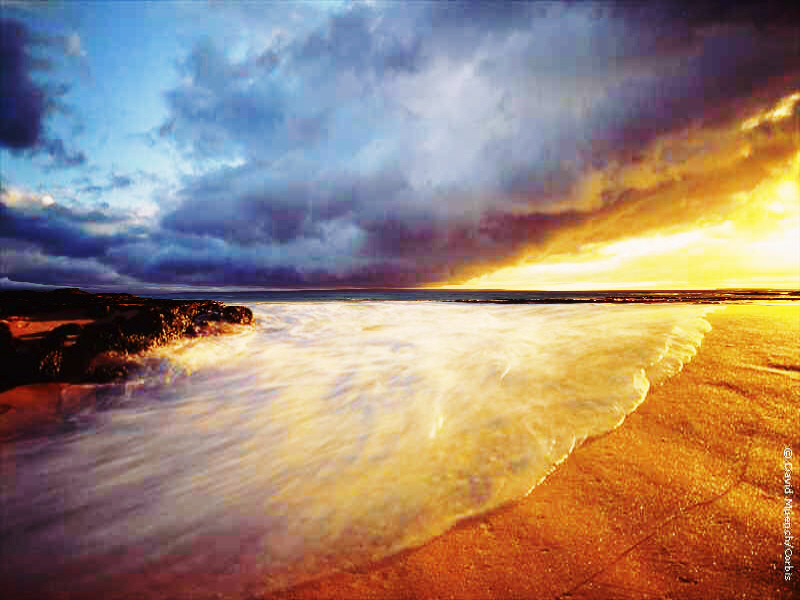﻿ FreePlaneBendCommand Class | Leadtools.ImageProcessing.SpecialEffects | Raster, Medical, Document Help
←Select platform
In This Topic ▼

# FreePlaneBendCommand Class

Summary

Wraps the image onto a 3D plane shaped by Curve. This command is available in the Imaging Pro and above toolkits.

Syntax
C#
VB
C++
``public class FreePlaneBendCommand : RasterCommand ``
````  `
`Public Class FreePlaneBendCommand  `
`   Inherits RasterCommand ````
``public ref class FreePlaneBendCommand : public RasterCommand   ``

Remarks
• If the image has a region, the effect will be applied on the region only.
• For an example, the following figure shows an image before the effect is applied.The following figure shows the same image after the effect has been applied.• This command supports 12-bit and 16-bit grayscale and 48-bit and 64-bit color images. Support for 12-bit and 16-bit grayscale and 48-bit and 64-bit color images is available only in the Document/Medical toolkits.

• This command does not support signed data images.
• This command does not support 32-bit grayscale images.

Example

Run the FreePlaneBendCommand on an image.

C#
VB
````using Leadtools; `
`using Leadtools.Codecs; `
`using Leadtools.ImageProcessing.SpecialEffects; `
`using Leadtools.ImageProcessing.Effects; `
` `
`public void FreePlaneBendCommandExample() `
`{ `
`   // Load an image `
`   RasterCodecs codecs = new RasterCodecs(); `
`   codecs.ThrowExceptionsOnInvalidImages = true; `
` `
`   RasterImage image = codecs.Load(Path.Combine(LEAD_VARS.ImagesDir, "sample5.cmp")); `
` `
`   // Prepare the command `
`   FreePlaneBendCommand command = new FreePlaneBendCommand(); `
`   LeadPoint[] UserPoint = new LeadPoint; `
`   int[] Curve = new int; `
` `
`   //Apply the filter `
`   UserPoint.X = 0; `
`   UserPoint.Y = 0; `
`   UserPoint.X = 3; `
`   UserPoint.Y = 10; `
`   UserPoint.X = 9; `
`   UserPoint.Y = -10; `
`   UserPoint.X = 12; `
`   UserPoint.Y = 0; `
` `
`   EffectsUtilities.GetCurvePoints(Curve, UserPoint, CurvePointsType.Linear); `
`   for (int i = 0; i < Curve.Length; i++) `
`      Curve[i] /= 50; `
`   command.Flags = FreePlaneBendCommandFlags.Color | FreePlaneBendCommandFlags.Vertical; `
`   command.Scale = 200; `
`   command.FillColor = new RasterColor(255, 0, 0); `
`   command.Curve = Curve; `
`   command.Run(image); `
`   codecs.Save(image, Path.Combine(LEAD_VARS.ImagesDir, "Result.jpg"), RasterImageFormat.Jpeg, 24); `
` `
`} `
` `
`static class LEAD_VARS `
`{ `
`   public const string ImagesDir = @"C:\Users\Public\Documents\LEADTOOLS Images"; `
`} ````
````Imports Leadtools `
`Imports Leadtools.Codecs `
`Imports Leadtools.ImageProcessing.SpecialEffects `
`Imports Leadtools.ImageProcessing.Effects `
` `
`Public Sub FreePlaneBendCommandExample() `
`   Dim codecs As New RasterCodecs() `
`   codecs.ThrowExceptionsOnInvalidImages = True `
` `
`   Dim leadImage As RasterImage = codecs.Load(Path.Combine(LEAD_VARS.ImagesDir, "sample5.cmp")) `
` `
`   ' Prepare the command `
`   Dim command As FreePlaneBendCommand = New FreePlaneBendCommand `
`   Dim UserPoint() As LeadPoint `
`   ReDim UserPoint(3) `
` `
`   Dim Curve() As Integer `
`   ReDim Curve(12) `
` `
`   'Apply the filter `
`   UserPoint(0).X = 0 `
`   UserPoint(0).Y = 0 `
`   UserPoint(1).X = 3 `
`   UserPoint(1).Y = 10 `
`   UserPoint(2).X = 9 `
`   UserPoint(2).Y = -10 `
`   UserPoint(3).X = 12 `
`   UserPoint(3).Y = 0 `
` `
`   EffectsUtilities.GetCurvePoints(Curve, UserPoint, CurvePointsType.Linear) `
`   command.Flags = FreePlaneBendCommandFlags.Color Or FreePlaneBendCommandFlags.Vertical `
`   command.Scale = 200 `
`   command.FillColor = New RasterColor(255, 0, 0) `
`   command.Curve = Curve `
`   command.Run(leadImage) `
`   codecs.Save(leadImage, Path.Combine(LEAD_VARS.ImagesDir, "Result.jpg"), RasterImageFormat.Jpeg, 24) `
` `
`End Sub `
` `
`Public NotInheritable Class LEAD_VARS `
`   Public Const ImagesDir As String = "C:\Users\Public\Documents\LEADTOOLS Images" `
`End Class ````

Requirements

Help Version 20.0.2020.3.31